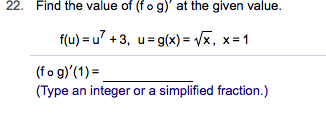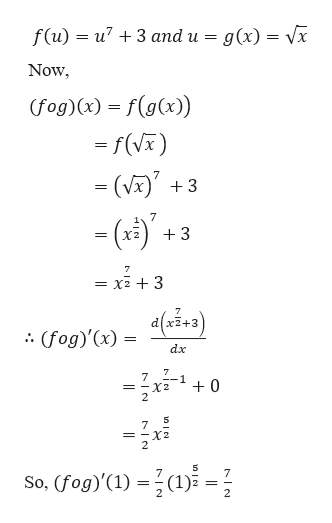22.Find the value of (f o g)' at the given valuef(u) u+3, u g(x)= /x. x=1(fo g)'(1)(Type an integer or a simplified fraction.)

Question

Can you help me step by step with this problem?help_outlineImage Transcriptionclose22. Find the value of (f o g)' at the given value f(u) u+3, u g(x)= /x. x=1 (fo g)'(1) (Type an integer or a simplified fraction.) fullscreen
Step 1

Given,

...help_outlineImage Transcriptionclosef(u)u73 and u g(x) = Vx Now (fog)(x) f(g(x)) -f(V) (V 3 = 7 3 ха 7 x23 = dlx2+3 (fog)'(x) = .. dx 7 -1 = -X2 2 0 7 = -X2 2 So, (fog)'(1) (1) 7 2 fullscreen

Want to see the full answer?

See Solution

Want to see this answer and more?

Our solutions are written by experts, many with advanced degrees, and available 24/7

See Solution
Tagged in

Calculus